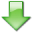Analytical argumentations of probability and statistics. Summary Some fundamental concepts of probability and statistics are treated, with the aim to circumstantiate and to establish some procedures of numerical elaboration. For this purpose deductive argumentations are used, performed in terms of logic and mathematical analysis. A wide attention to the preliminary description of the logical and mathematical symbolic tools is dedicated. Are extensively shown fundamental properties and definitions, pertaining to the concepts of event and probability. Is reached the probability density of a random variable, by means of the analysis of properties characteristic of statistical sample and universe. Are presented important aspects of the probability density of a set and of a function of random variables. Essential properties are deduced, whom the probability density the expected value and the variance, of the random variables more used. The statistical test and the inherent hypotheses are treated, widely and in details, and lower bounds for the probability of these are reached. The conditions are shown in order to determine lower and upper bounds for the probability that an unknown quantity is found in one given zone of the real axis. Finally the analysis of variance and the regression analysis are treated, with many particulars and in the generally multidimensional aspect. Keywords: probability, statistics, mathematical analysis, mathematical logic, numerical elaboration. INDEX 1  INTRODUCTION 2  PRELIMINARY COGNITIONS. 2.1  Notions of mathematical logic. 2.1.1  Propositions and deductions. 2.1.2  Other symbolic conventions. 2.2  Notions of set theory. 2.3  Notions of linear algebra. 2.4  Notions of mathematical analysis. 2.4.1  The sets of real numbers, and the multidimensional Euclidean space. 2.4.2  The analytic functions. 2.4.3  The independence between variables. 2.4.4  The derivatives and the integrals. 2.4.5  The minimum and maximum value of a function. 2.4.6  The one-to-one correspondence between variables. 2.4.7  The square root, the quadratic equation, the quadratic form, the weighted average. 3  THE EVENTS AND THE PROBABILITY. 3.1  The events. 3.2  The probability. 3.2.1  Some remarkable expressions. 4  THE PROBABILITY DENSITY OF A RANDOM VARIABLE. 4.1  The sample, the population, the relative frequency. 4.2  The statistical estimator, the probability density, the random variable. 5  THE RANDOM VARIABLES SETS AND FUNCTIONS. 5.1  The joint probability density. 5.2  The random variables functions probability density. 5.2.1  Two expressions of general validity. 5.2.2  The one-to-one correspondence between random variables. 5.2.3  The sum, the product, the quotient, the square of random variables. 5.2.4  The random variable expressed from a quadratic function. 5.3  The expected value and the variance of a random variables function. 5.3.1  The Chebyshev's inequality, and the law of large numbers. 5.3.2  The functions, moment-generating and characteristic, of a random variable. 6  THE PROBABILITY DENSITY OF SOME BETWEEN THE RANDOM VARIABLES MORE NOTES. 6.1  The uniform random variable. 6.1.1  The determination of a random sample of the universe of the values of a random variable. 6.2  The normal (or Gaussian) variable. 6.2.1  The central limit theorem. 6.2.2  The Montecarlo method for the approximation of an integral. 6.3  The chi-square variable. 6.4  The t-Student and F-Fisher variables. 7  THE STATISTICAL TEST. 7.1  The preliminary positions. 7.2  The hypotheses inherent a statistical test. The probabilities of making two remarkable errors, sayings respectively of type I and II. 7.3  The execution of a statistical test. 7.4  The truth probability for the hypotheses inherent a statistical test. 8  THE CONFIDENCE REGION FOR AN UNKNOWN QUANTITY. 8.1  The confidence region of four remarkable unknown quantity, inherent the normal probability density function. 8.1.1  The expected value of a random variable. 8.1.2  The variance of a random variable. 8.1.3  The mean value of a set of random variables. 8.1.4  The variance of a set of random variables. 9  THE ANALYSIS OF THE VARIANCE. 9.1  The tabular structure and his meanings. 9.2  The statistical estimators, the hypothesis, and the consequent probability density functions. 9.3  The statistical tests that constitute the analysis of the variance. 10  THE REGRESSION ANALYSIS. 10.1  The least squares polynomial, and the statistical estimators inherent. 10.2  The hypotheses on the sample, and the consequent probability density functions. 10.3  The regression equation, the other hypotheses of it and of the sample, the statistical tests. 10.4  The other results of the regression analysis. 10.4.1  The probability of a hypothesis of specific type on sample and universe. 10.4.2  The confidence regions for some quantities of greater relief, and other remarkable probabilities. CONCLUSIONS BIBLIOGRAPHY Date of release:  Tuesday 13 January 2007 Language: italian Number of downloads (from 28 June 2008): 7617THE FULL TEXT as a 3248 kilobytes PDF file (can be opened by the free Adobe Reader 6.0 and later) Related links:  Argomentazioni analitiche di probabilità e statistica, Aracne Editrice (Scienze matematiche e informatiche), febbraio 2013, ISBN: 978-88-548-5831-2 The Probability Web - Teaching Resources Open Directory Project - Science/Math/Probability/Teaching_Resources Open Directory Project - World/Italiano/Scienza/Matematica The Math Forum of the Drexel University School of Education